# Tutorial: Relativity, Cosmic Rays, and Accelerators

## Some special relativity

If you have measure muon time of flight, you can calculate the speed of the muon and the Lorentz factor for the muons.

For example, if the distance the muons travel is, say, d = 10 m and the TOF is measured as t = 34.0 nanoseconds, then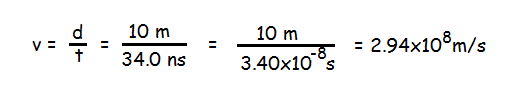The fraction of the speed of light "beta" is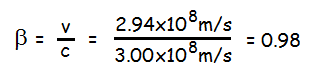The Lorentz factor "gamma" is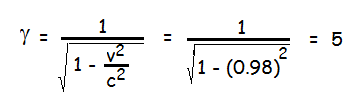Another way to get "gamma" for a particle is to use the energy and mass relationship

In our example, we can use E = γmc2 to find the energy of our muon, since we know the invariant mass of a muon is m = 106 MeV/c2: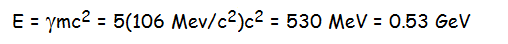## Application to accelerators

The ILC will be 30-50 km long and energize electrons and positrons to 250 GeV or more so that they will collide with energies upwards of 500 GeV: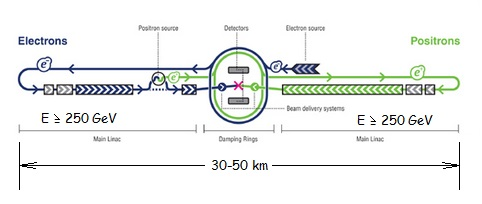Imagine we want to build a muon-antimuon collider about the size and energy of the ILC. We might want to do this to make a smaller collider with similar properties, because:

1. Muon-antimuon and electron-positron collisions yield substantially the same results. Both are leptons with no structure we can detect, meaning the entire energy of the collion can go into creating one particle or particle pair, leaving no background.
2. Muons are much more massive. The ratio of masses is mμ/me = (106 MeV/c2)/(0.511 MeV/c2) = 207. This means, in accelerating, they yield much less energy per unit mass and, therefore, it is more practical to build a circular collider (like the LHC) with counter-rotating beams. This saves space and makes it easier to build.
3. In the LHC, the circulating beam allows physicists to collide the same bunches of beam particles again and again, so they do not have to be continually made and accelerated. A "fill" of beam particles in the accelerator can last up to 24 hours.

So, why not build a muon collider? Some people are working on this concept, most notably in the MICE experiment

However, there is one other fact that is important to this: the lifetime of the muon is approximately 2.18 μs.